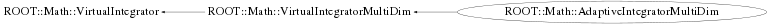# class ROOT::Math::AdaptiveIntegratorMultiDim: public ROOT::Math::VirtualIntegratorMultiDim

```
class for adaptive quadrature integration in multi-dimensions using rectangular regions.
Algorithm from  A.C. Genz, A.A. Malik, An adaptive algorithm for numerical integration over
an N-dimensional rectangular region, J. Comput. Appl. Math. 6 (1980) 295-302.

Converted/adapted by R.Brun to C++ from Fortran CERNLIB routine RADMUL (D120)
The new code features many changes compared to the Fortran version.

Control  parameters are:

minpts: Minimum number of function evaluations requested. Must not exceed maxpts.
if minpts < 1 minpts is set to 2^n +2*n*(n+1) +1 where n is the function dimension
maxpts: Maximum number of function evaluations to be allowed.
maxpts >= 2^n +2*n*(n+1) +1
if maxpts<minpts, maxpts is set to 10*minpts
epstol, epsrel   : Specified relative and  absolute accuracy.

The integral will stop if the relative error is less than relative tolerance OR the
absolute error is less than the absolute tolerance

The class computes in addition to the integral of the function is the desired interval:

an estimation of the relative accuracy of the result.
number of function evaluations performed.
status code  :
0 Normal exit.  . At least minpts and at most maxpts calls to the function were performed.
1 maxpts is too small for the specified accuracy eps.
The result and relerr contain the values obtainable for the
specified value of maxpts.
3 n<2 or n>15

Method:

An integration rule of degree seven is used together with a certain
strategy of subdivision.
For a more detailed description of the method see References.

Notes:

1.Multi-dimensional integration is time-consuming. For each rectangular
subregion, the routine requires function evaluations.
Careful programming of the integrand might result in substantial saving
of time.
2.Numerical integration usually works best for smooth functions.
Some analysis or suitable transformations of the integral prior to
numerical work may contribute to numerical efficiency.

References:

1.A.C. Genz and A.A. Malik, Remarks on algorithm 006:
An adaptive algorithm for numerical integration over
an N-dimensional rectangular region, J. Comput. Appl. Math. 6 (1980) 295-302.
2.A. van Doren and L. de Ridder, An adaptive algorithm for numerical
integration over an n-dimensional cube, J.Comput. Appl. Math. 2 (1976) 207-217.

@ingroup Integration

```

## Function Members (Methods)

public:
 virtual ~AdaptiveIntegratorMultiDim() ROOT::Math::AdaptiveIntegratorMultiDim AdaptiveIntegratorMultiDim(const ROOT::Math::AdaptiveIntegratorMultiDim&) ROOT::Math::AdaptiveIntegratorMultiDim AdaptiveIntegratorMultiDim(double absTol = 1.E-9, double relTol = 1E-9, unsigned int maxpts = 100000, unsigned int size = 0) ROOT::Math::AdaptiveIntegratorMultiDim AdaptiveIntegratorMultiDim(const ROOT::Math::IMultiGenFunction& f, double absTol = 1.E-9, double relTol = 1E-9, unsigned int maxcall = 100000, unsigned int size = 0) virtual double Error() const virtual double Integral(const double* xmin, const double* xmax) double Integral(const ROOT::Math::IMultiGenFunction& f, const double* xmin, const double* xmax) virtual int NEval() const ROOT::Math::VirtualIntegratorMultiDim& ROOT::Math::VirtualIntegratorMultiDim::operator=(const ROOT::Math::VirtualIntegratorMultiDim&) virtual ROOT::Math::IntegratorMultiDimOptions Options() const double RelError() const virtual double Result() const virtual void SetAbsTolerance(double absTol) virtual void SetFunction(const ROOT::Math::IMultiGenFunction& f) void SetMaxPts(unsigned int n) void SetMinPts(unsigned int n) virtual void SetOptions(const ROOT::Math::IntegratorMultiDimOptions& opt) virtual void SetRelTolerance(double relTol) void SetSize(unsigned int size) virtual int Status() const virtual ROOT::Math::IntegrationMultiDim::Type ROOT::Math::VirtualIntegratorMultiDim::Type() const
protected:
 double DoIntegral(const double* xmin, const double* xmax, bool absVal = false)

## Data Members

private:
 double fAbsTol absolute tolerance unsigned int fDim dimentionality of integrand double fError integration error const ROOT::Math::IMultiGenFunction* fFun pointer to integrand function unsigned int fMaxPts maximum number of function evaluation requested unsigned int fMinPts minimum number of function evaluation requested int fNEval number of function evaluation double fRelError Relative error double fRelTol relative tolerance double fResult last integration result unsigned int fSize max size of working array (explode with dimension) int fStatus status of algorithm (error if not zero)

## Class Charts## Function documentation

AdaptiveIntegratorMultiDim(double absTol = 1.E-9, double relTol = 1E-9, unsigned int maxpts = 100000, unsigned int size = 0)
```      construct given optionally tolerance (absolute and relative), maximum number of function evaluation (maxpts)  and
size of the working array.
The size of working array represents the number of sub-division used for calculating the integral.
Higher the dimension, larger sizes are required for getting the same accuracy.
The size must be larger than  >= (2N + 3) * (1 + MAXPTS/(2**N + 2N(N + 1) + 1))/2). For smaller value passed, the
minimum allowed will be used

```
AdaptiveIntegratorMultiDim(const ROOT::Math::IMultiGenFunction& f, double absTol = 1.E-9, double relTol = 1E-9, unsigned int maxcall = 100000, unsigned int size = 0)
```      Construct with a reference to the integrand function and given optionally
tolerance (absolute and relative), maximum number of function evaluation (maxpts)  and
size of the working array.

```
```      destructor (no operations)

```
`{}`
double Integral(const double* xmin, const double* xmax)
```      evaluate the integral with the previously given function between xmin[] and xmax[]

```
return DoIntegral(const double* xmin, const double* xmax, bool absVal = false)
double Integral(const ROOT::Math::IMultiGenFunction& f, const double* xmin, const double* xmax)
``` evaluate the integral passing a new function
```
void SetFunction(const ROOT::Math::IMultiGenFunction& f)
``` set the integration function (must implement multi-dim function interface: IBaseFunctionMultiDim)
```
double Result() const
``` return result of integration
```
`{ return fResult; }`
double Error() const
``` return integration error
```
`{ return fError; }`
double RelError() const
``` return relative error
```
`{ return fRelError; }`
int Status() const
``` return status of integration
```
`{ return fStatus; }`
int NEval() const
``` return number of function evaluations in calculating the integral
```
`{ return fNEval; }`
void SetRelTolerance(double relTol)
``` set relative tolerance
```
void SetAbsTolerance(double absTol)
``` set absolute tolerance
```
void SetSize(unsigned int size)
```set workspace size
```
`{ fSize = size; }`
void SetMinPts(unsigned int n)
```set min points
```
`{ fMinPts = n; }`
void SetMaxPts(unsigned int n)
```set max points
```
`{ fMaxPts = n; }`
void SetOptions(const ROOT::Math::IntegratorMultiDimOptions& opt)
``` set the options
```